# Numbers - math word problems

#### Number of problems found: 1462

• Boys and girlsThere are 20 boys and 10 girls in the class. How many different dance pairs can we make of them?
• Sum and roundingI know two numbers whose sum is 20. When they each round and then added together I get the 30. What are this numbers?
• Seven timesWhich number seven times is just as higher as 27, how much is smaller than 29?
• Sum of inner angles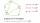Prove that the sum of all inner angles of any convex n-angle equals (n-2) . 180 degrees.
• CircleWrite the equation of a circle that passes through the point [0,6] and touch the X-axis point [5,0]: ?
• Pimps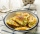There were 24 pimps in the plate. Maros ate 12, his little sister four times less. How many pimps remained in the plate?
• Fill by colour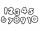Write numbers from 0 to 38 on the paper. If the two numbers differ by 2, color them same colour. How many pairs do you colour?
• Dance ensemble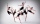The dance ensemble took the stage in pairs. During dancing, the dancers gradually formed groups of four, six and nine. How many dancers have an ensemble?
• Farm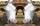Agricultural cooperative (farm) produced 14144 liters of milk for 16 days. Which exceeded the plan by 1984 liters. How many liters of milk a day had planned to produce?
• Estate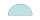Semicircle estate must be fence. The straight section has 26 meters long fence. How many meters of fence should buy?
• JagdeepJagdeep had a strip of paper measuring 1.8 m. He cut it into 4 equal strips. How long is each shorter strip of paper?
• GardenHow many steps of 76 cm circumvent square garden with area 1.8 ha?
• 120 nuts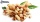Divide 120 nuts in a ratio of 4: 6.
• Lcm simpleFind least common multiple of this two numbers: 140 175.
• Parenthesis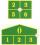Fill in the examples correctly brackets: A) 7.9+12:3-2 =23 B) 7.9+12:3-2=75
• How manyHow many different rectangles with integer page lengths have an area S = 60 cm²?
• Rolls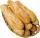Mom bought 13 rolls. Dad ate 3.5 rolls. How many rolls left when Peter yet put two at dinner?
• SummandOne of the summands is 145. The second is 10 more. Determine the sum of the summands.
• Large familyI have as many brothers as sisters and each my brother has twice as many sisters as brothers. How many children do parents have?
• Wire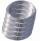From wire 53 meters long they cut of 12.1 m and then 13.1 m. How many meters of wire left?

Do you have an interesting mathematical word problem that you can't solve it? Submit a math problem, and we can try to solve it.

We will send a solution to your e-mail address. Solved examples are also published here. Please enter the e-mail correctly and check whether you don't have a full mailbox.

Please do not submit problems from current active competitions such as Mathematical Olympiad, correspondence seminars etc...